# TANCET 2013 Quant Qn 3: Indices

## Question

Medium Quantitative Reasoning

If ( 0.004 * 10a )( 0.32 * 10b ) = 128 * 103, a + b = ?

1. 3
2. 5
3. 6
4. 8
5. -2

Correct Answer    Choice 4. a + b = 8

• ### Step by step solution

1. (0.004 * 10a)(0.32 * 10b) = 128 * 103
2. We can write 0.004 as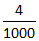Similarly, we can write 0.32 as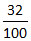3. So, the expression can be rewritten as (* 10a)(* 10b) = 128 * 103

4. Or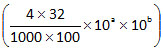= 128 * 103

5. i.e.,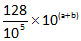= 128 * 103

6. i.e., 128 * 10a + b - 5 = 128 * 103

7. Dividing both sides by 128, we get 10a + b - 5 = 103

8. As the bases are equal, we can equate the indices.
a + b - 5 = 3
Or a + b = 8

The correct answer is Choice 4. (a + b) = 8

• ### Remember these Rules of Indices

1. ax * ay = ax + y

2. (ax)y = axy

3.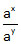= ax - y

4.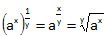e.g.,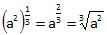5. a-x =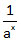6. ax * bx = (a*b)x

## Online TANCET MBA CourseTry it Free!

Register in 2 easy steps and start learning in 5 minutes!